# JEE Advanced Question Paper 2019 Physics Paper 2

JEE Advanced 2019 Question Paper 2 with solutions for Physics is available here. All questions are solved by subject experts and in a detailed manner. Listed solutions can also be downloaded in a PDF format for offline use. Practising JEE Advanced 2019 Physics Question Paper 2 will help the aspirants to study productively and at the same time understand the coverage of topics as well as the overall exam pattern for exam. To succeed in the JEE Advanced upcoming exams candidates are advised to practice sample papers to prepare well and effectively.

### Paper 2 - Physics

Question 1: Consider two plane convex lens of same radius of curvature and refractive index n1 and n2 respectively. Now consider two cases: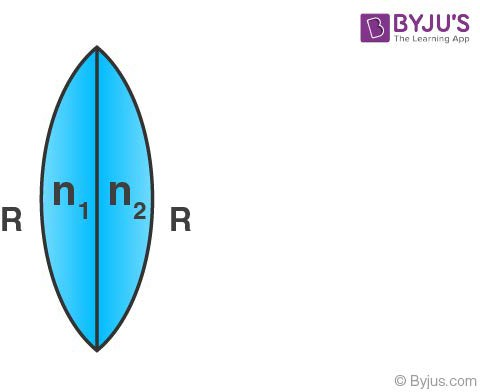Case – I: When n1 = n2 = n, then equivalent focal length of lens is f0.

Case – II: When n1 = n, n2 = n + Δn, then equivalent focal length of lens is f= f0 + Δf0 . Then correct options are:

1. a) If Δn/n > 0, then Δf0/f0 < 0
2. b) |Δf0/f0| < |Δn/n|
3. c) If n = 1.5, Δn = 10-3 and f0 = 20 cm then |Δf0= 0.02 cm
4. d) If Δn/n < 0, then Δf0/f0 > 0

Solution:

1. $$\frac{1}{f_1} = (n-1)(\frac{1}{f}) => \frac{1}{f_0} = \frac{2(n-1)}{R}$$
…..(1)

$$\frac{1}{f_2} = (n+ \Delta n -1)(\frac{1}{R} - \frac{1}{\infty})$$

$$\frac{1}{f + \Delta f_0} = \frac{n -1}{R} + (n + \Delta n - 1)(\frac{1}{f})$$

=

$$\frac{2n + \Delta n - 2}{R}$$
….(2)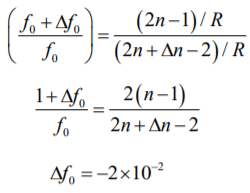Question 2: In YDSE monochromatic light of wavelength 600 nm incident of slits as shown in figure.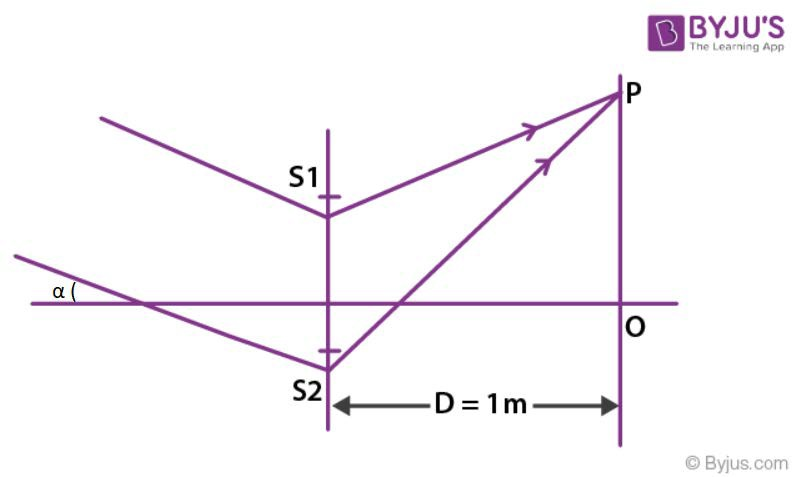If S1S2 = 3mm, OP = 11 mm then

1. a) If α = 0.36/π degree then destructive interfaces at point P.
2. b) If α = 0.36/π degree then constructive interfaces at point O.
3. c) If α = 0 then constructive interfaces at O
4. d) Fringe width depends an α

Solution:

1. Δx = d sin α + d sin θ

= dα + dy/D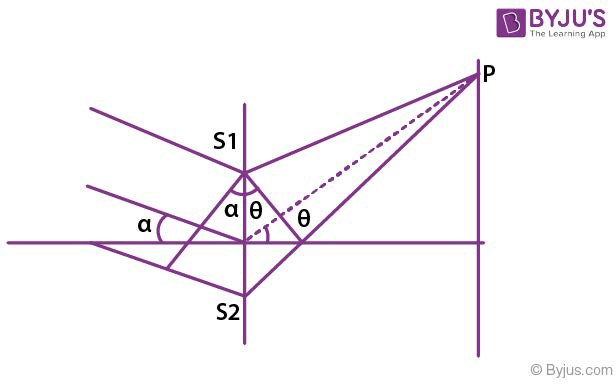(A)

$$\Delta x = 3 \times 10^{-3} \times \frac{.36}{\pi} \times \frac{\pi}{180} + \frac{3 \times 11 \times 10^{-6}}{1} = 3900$$

3900 = (2n – 1) λ/2 => n = 7 dest

(B) Δx = 3mm (.36/π) (π/180) = 600 nm

600nm = n600nm

=>n = 1 const

(C) α = 0, Δx = 0

So constructive interference

(D) Fringe width does not depend on alpha;.

Question 3: A uniform rigid rod of mass m & length l is released from vertical position on rough surface with sufficient friction for lower end not to slip as shown in figure. When rod makes angle 60° with vertical then find correct alternatives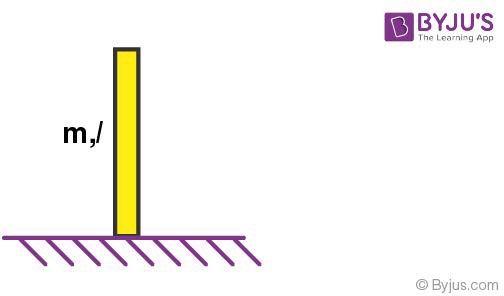1. a)
$$\alpha = \frac{2g}{l}$$
2. b)
$$\sqrt{\frac{3g}{2l}}$$
3. c) N = mg/16

Solution:

1.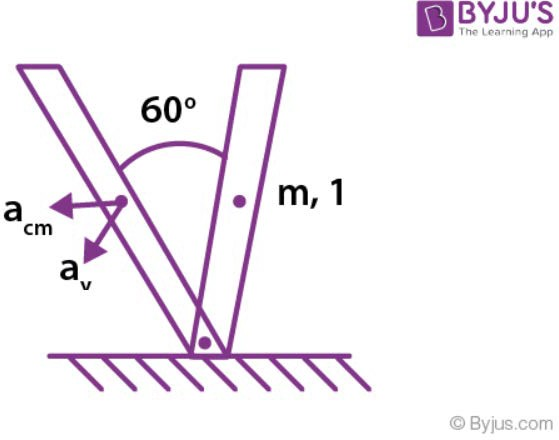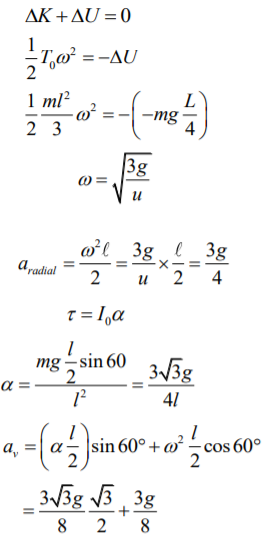mg – N = mav

N = mg/16

Question 4: Monoatomic gas A having 5 mole is mixed with diatomic gas B having 1 mole in container of volume V0. Now the volume of mixture is compressed to V0/4 by adiabatic process. Initial pressure and temperature of gas mixture is P0 and T0. [given 23.2 = 9.2] Choose correct option:

1. a)
$$\gamma_{mix} = 1.6$$
2. b) Final pressure is between 9P0 and 10P0
3. c) |W.D.| = 13 RT0
4. d) Average Translational kinetic energy

Solution:

1. $$\gamma_{mix} = \frac{n_1C_{P_1} + n_2 l_{P_2}}{n_1C_{V_1} + n_2 C_{V_2}} =\frac{8}{5}$$

W = [P1V1 – P2V2]/[V-1]

P0V08/5 = P2 (V0/9)8/5

P2 = 9.2 P0

$$\omega = \frac{P_0V_0- 92P_0V_0/4}{3/5}=-13RT_0$$

Question 5: The given arrangement is released from rest when spring is in natural length. Maximum extension in spring during the motion is x0, a1, a2 and a3 are accelerations of the blocks.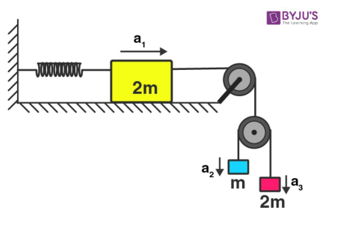Make the correct options

1. a) a2 – a1 = a1 – a3
2. b) x0 = 4mg/3k
3. c)
$$v = \frac{x_0}{2}\sqrt{\frac{3k}{14m}}$$
4. d) Acceleration a1 at x0/4 is 3kx0/42m

Solution:

1. 2a1 = a2 + a3

a1 - a3 = a2 - a1

When we use m equivalent (for other opitions)

T/g' = 2(2m)m/(2m+m) = 4m/3

2T/g' = 8m/3

meq. = 8m2/[m+2m] = 8m/3

(1/2) kx02 = 8mgx0/3

x0 = 16mg/3k

Vx0/2 = Vmax = x0w/2

=

$$\frac{x_0}{2} \sqrt{\frac{k}{2m + \frac{8m}{3}}} = \frac{x_0}{2} \sqrt{\frac{3k}{14m}}$$

ax0/4 = x0w2/4 = x0/4 * 3k/14m = 3kx0/42m

Question 6: A dipole of Dipole moment

$$p = \frac{p_0}{\sqrt 2}(\hat i + \hat j)$$
is placed at origin. Now a uniform external electric field at magnitude E0 is applied along direction of dipole. Two points A and B are lying on a equipotential surface of radius R centered at origin. A is along axial position of dipole and B is along equatorial position. There correct option are: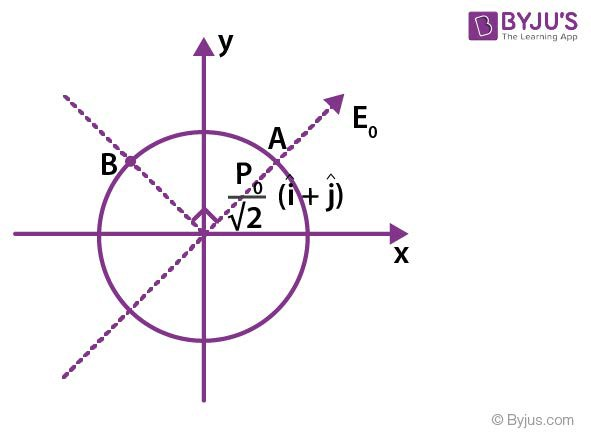1. a) Net electric field at point A is 3E0
2. b) Net electric field at point B is Zero
3. c) Radius of equatorial surface R = (kp0/E0)1/3
4. d) Radius of equatorial surface R = (√2kp0/E0)1/3

Solution:

1.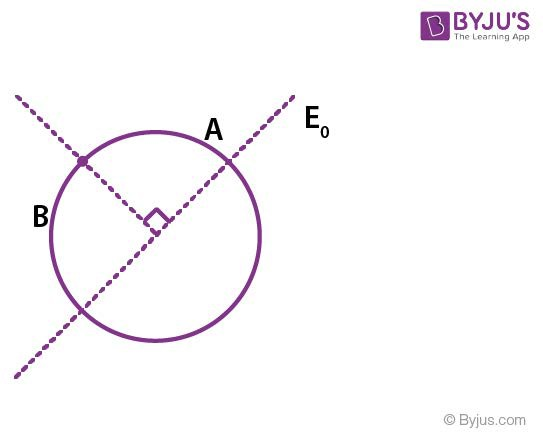P = (P0/√2) (x + 1)

KP0/r3 = E0

(EA)net = 2KP0/r3 + E0 = 3E0

(EA)net = 0

Question 7: A free hydrogen atom after absorbing a photon of wavelength λa gets excited from state n = 1 to n = 4. Immediately after electron jumps to n = m state by emitting a photon of wavelength λe. Let change in momentum of atom due to the absorption and the emission are ΔPa and Δpe respectively. If λa/ λe = 1/5.

Which of the following is correct

1. a) m = 2
2. b) ΔPa/Pe = 1/2
3. c) λe = 418 nm
4. d) Ratio of K.E. of electron in the state n = m to n = 1 is ¼.

Solution:

1.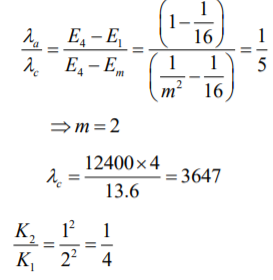Question 8: In a cylinder a heavy piston is moving with speed v as shown diagram and gas is filled inside it. A gas molecule is moving with speed v0 towards moving piston. Then which of the following is

correct (Assume v <<<< v0 Δl/l ) and collision is elastic)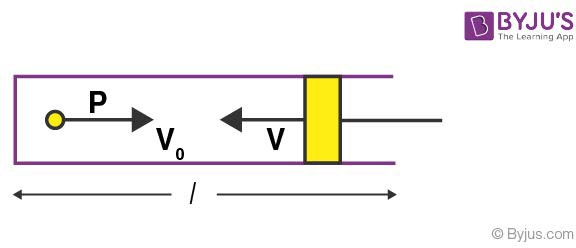1. a) change in speed after collision is 2V
2. b) change is speed after collision is 2 v0 Δl/l
3. c) rate of collision is V/l
4. d) When piston is at l/2 its kinetic energy will be four times.

Solution:

1.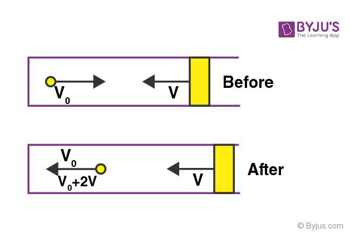Change in speed is (2V + V0 – V0) = 2V

Question 9: Calculate the work done if a particle is moved along a path AB−BC−CD−DE−EF−FA, as shown in the figure in presence of a force

$$\vec{F} = (\alpha y\hat{ii} + 2\alpha x\hat{j})N$$
, where x and y are in meter and
$$\alpha w = -1Nm^{-1}$$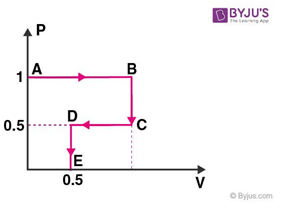Solution:

1. dω = αy dx + 2αx dx

$$\omega_{A \rightarrow B}= \int \alpha y dx = \alpha 1\int_0^1 dx = \alpha$$

$$\omega_{B \rightarrow C}= 2 \alpha 1\int_1^{0.5} dy = -\alpha$$

$$\omega_{C \rightarrow D}= \int_1^{0.5} \alpha y dx = -\alpha/4$$

$$\omega_{D \rightarrow E}= 2 \alpha \int_{0.5}^0 x dy = -\alpha/2$$

E → F, y = 0, dy = 0, W<sub>EF</sub> = 0

F → A, x = 0, dx = 0, W<sub>F → A</sub> = 0

∴ w = α − α − α / 4 − α / 2 = -3α / 4

Given α = −1

⇒ W = + 3/4 J = 0.75 J.

Question 10: In a given circuit inductor of L = 1mH and resistance R = 1Ω are connected in series to ends of two parallel conducting rods as shown. Now a rod of length 10 cm is moved with constant velocity of 1 cm/s in magnetic field B = 1T. If rod starts moving at t = 0 then current in circuit after 1 millisecond is x . 10-3 A. Then value of x is: (given e-1 = 0.37)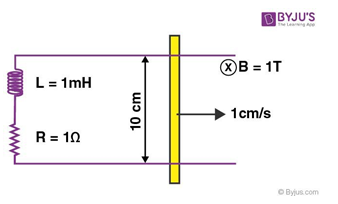Solution:

1. e = (V x B) dl = 10-3 v df

i = 10-3(1 – e-1)

i = 0.63 mA

Question 11: A prism is shown in the figure with prism angle 75° and refractive index √3. A light ray incidents on a surface at incident angle θ. Other face is coated with a medium of refractive index n. For θ ≤ 600 ray suffers total internal reflection find value of n2.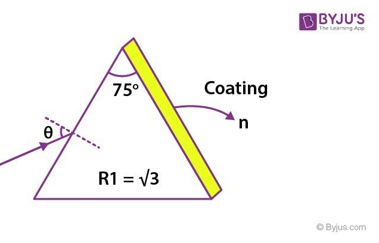Solution:

1.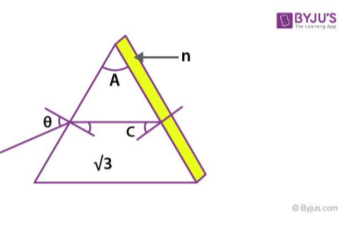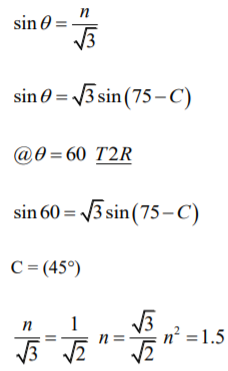Question 12: Perfectly reflecting mirror of mass M mounted on a spring constitute a spring mass system of angular frequency Ω such that [4πMΩ]/h = 1024 m-2 where h is plank constant. N photons of wavelength λ = 8π x 10-6 m strikes the mirror simultaneously at normal incidence such that the mirror gets displaced by 1 μm. If the value of N is x * 1012, then find the value of x.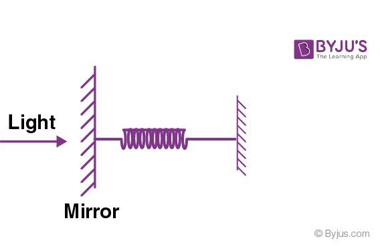Solution:

1. Photons are reflected

MV = 2Nh/λ [mean]

Vmean = αA

A = 1 min

N =

$$\frac{m \alpha 10^{-6} \lambda}{2h}$$

N = 4Mπα/h x 10-12

1024 x 10-12

1 x 10-12

Therefore, X = 1

Question 13: The sample of monoatomic gas undergoes a process as represented by P – V graph (if P0V0 = 1/3 RT0) then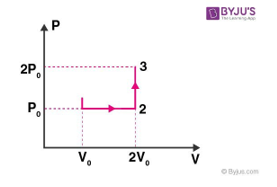(P) W1->2 = 1/3 RT0 (Q) Q1->2->3 = 11/6 RT0 (R) U1->2 = RT0/2 (S) W1->2->3 = 1/3 RT0

Which of the following options are correct

1. a) P, Q, R, S are correct
2. b) Only P, Q are correct
3. c) Only R, S are correct
4. d) Only P, R, S correct

Solution:

1.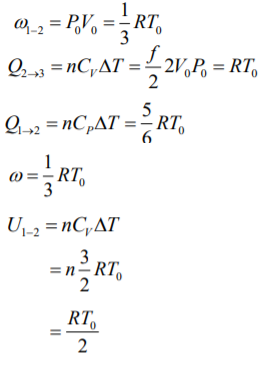Question 14: The sample of monoatomic gas undergoes a process as represented by T – V graph (if P0V0 = 1/3 RT0) then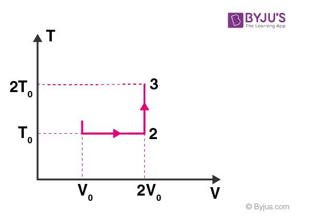(P) W1->2 = 1/3 RT0 ln 2

(Q) Q1->2->3 = 1/6 RT0 (2 ln (2) + 3 )

(R) U1->2 = 0

(S) W1->2->3 = [RT0/3] ln 2

Which of the following options are correct

1. a) P, Q are incorrect
2. b) R, S are incorrect
3. c) P, Q, S are incorrect
4. d) None of these

Solution:

1. ω1-2 = nRT0 ln 2

Q1-2-3 = Q12 + Q23

= d ω1-2 + dU2-3

= [RT0/3] ln 2 + n (f/2) RT0

= [RT0/3] ln 2 + (1/3) (3/2) RT0

U1-2=0

ω1-2-3 = (1/3) R0T0 ln 2

Question 15: Length of string of a musical instrument is varied from Lo to 2Lo in 4 different cases. Wire is made of different materials of mass per unit length μ, 2μ, 3μ, 4μ respectively. For first case (string – 1) length is Lo, Tension is To then fundamental frequency is fo, for second case length of the string is 3L0/2 (3rd Harmonic), for third case length of the string is 5L0/4 (5th Harmonic) and for the fourth case length of the string is 7L0/4 (14th harmonic). If frequency of all is same then tension in strings in terms of To will be:

1. a) String - 1 (P) T0
2. b) String – 2 (Q) T0/√2
3. c) String - 3 (R) T0/2
4. d) String - 4 (S) T0/16
(T) 3T0/16

Solution:

1.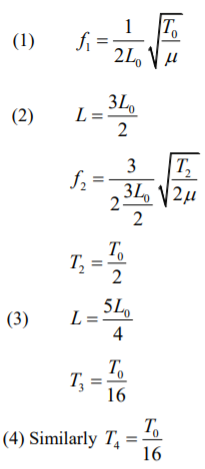(A) → P, (B) → R, (C) → T, (D) → S

Question 16: The free length of all four string is varied from L0 to 2L0. Find the maximum fundamental frequency of 1, 2, 3, 4 in terms of f0 (tension is same in all strings)

1. a) String – 1 (P) 1
2. b) String – 2 (Q) 1/2
3. c) String – 3 (R) 1/√2
4. d) String – 4 (S) 1/√3
(T) 1/16
(U) 3/16

Solution:

1.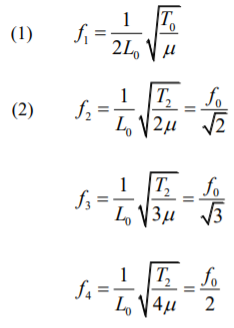(A) → P, (B) → R, (C) → S, (D) → Q

### JEE Advanced 2019 Physics Paper 2 Solutions